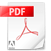## LOCKER PRANK RIDDLE AND SOLUTION

The Locker Prank Riddle

There are 100 lockers that line the main hallway of Cadillac High School. Every night, The school principal makes sure all the lockers are closed so that there will be an orderly start to the next day. One day, 100 mischievous students decide they will play a prank.

The students meet before school starts and line up. The first student then walks down the hallway and opens every locker. The next student follows by closing every other locker (starting at the second locker). Student three then goes to every third locker (starting with the third) and opens it if it's closed and closes it if it's open. Student four follows by opening every fourth locker if it's closed and closing it if it's open. This continues until student one hundred finally goes to the hundredth locker. When the principal arrives later in the morning, which lockers are open?

To determine the solution to the riddle, the key is to consider which students will open or shut any particular locker.

The Locker Prank Riddle Solution

The key here is to consider which students will open or shut any particular locker. Let’s take an example of locker 24. Student 1 will open it, since student 1 opens every locker. Student 2 will then close it, since student 2 closes every even locker and 24 is even. Student 3 will open it and then student 4 will shut it, since 24 is a multiple of both 3 and 4. Student 5 will pass it by since 24 is not a multiple of 5.

This example makes it clear that each student will change the status of all lockers that have a number that is a multiple of the student’s number. Conversely, every locker will have its status changed by the students that are numbered by one of the locker’s factors. Thus, locker 24 will have its status changed by students 1, 2, 3, 4, 6, 8, 12, and 24.

Now, how does this lead us to figure out which lockers are opened at the end? Locker 1, which has one factor, will clearly be open at the end, since the only student who touches it is the first student, who opens it. Locker 2, with two factors, will be closed, since the only two students to touch it are student 1, who opens it, and then student 2, who closes it.

Locker 3, also with two factors, will also be closed at the end. On the other hand, locker 4, which has three factors (1, 2, and 4), will be open, shut, and then open again. This line of thinking leads us to the conclusion: Only those lockers with an odd number of factors will be left open at the end of the prank.

So which numbers have an odd number of factors?

Consider that a factor is an integer that, when multiplied by another integer, produces the number of interest. Thus, factors are always paired with “another integer.” For instance, the factors of 24 are paired in the following way: (1, 24), (2, 12), (3, 8), (4, 6). Since factors come in pairs, most numbers have an even number of factors. The only exception occurs when factors are paired with themselves.

Let’s look at the factor “pairs” for 16: (1, 16), (2, 8), (4, 4). In this case, 4 is paired with itself to produce the number 16. Thus, 16 has an odd number of factors: 1, 2, 4, 8, 16. Factors are only ever paired with themselves in the case of perfect squares, which means that perfect squares are the only numbers with an odd number of factors! Therefore, the lockers that are left open are: 1, 4, 9, 16, 25, 36, 49, 64, 81, and 100.The Locker Prank Riddle and Solution